Levels »

# Ground Floor

### Demo 2.0, Elevation: 0.0m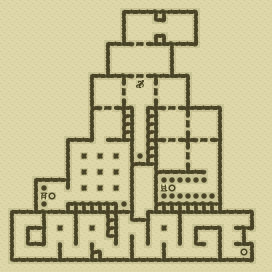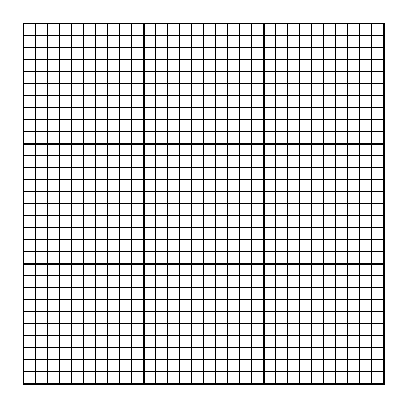### Items

•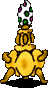Easter Egg (21, 1)
•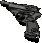Walther P4 Pistol (16, 9) (on Anonymous German)
•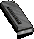6 Walther P4 Magazines (48 rounds) (16, 9) x8 (in Walther P4 Pistol) (on Anonymous German) - (16, 9) x8 (on Anonymous German) - (16, 9) x8 (on Anonymous German) - (16, 9) x8 (on Anonymous German) - (12, 10) x8 - (20, 10) x8
•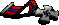Silver Medal (16, 9) (on Anonymous German)
•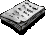Note (16, 11)
•Bungie Propaganda (16, 2)

•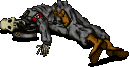(16, 9) Anonymous German
•Walther P4 Pistol
•Walther P4 Magazine x8
•Walther P4 Magazine x8
•Walther P4 Magazine x8
•Walther P4 Magazine x8
•Silver Medal

### Monsters

•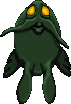29 Nightmares (17, 5) - (18, 5) - (15, 6) - (18, 7) - (6, 26) - (11, 26) - (14, 26) - (20, 26) - (22, 26) - (28, 26) - (29, 26) - (7, 27) - (11, 27) - (20, 27) - (22, 27) - (20, 28) - (22, 28) - (24, 28) - (25, 28) - (28, 28) - (16, 29) - (22, 29) - (25, 29) - (27, 29) - (27, 29) - (1, 30) - (9, 30) - (16, 30) - (29, 30)
•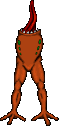20 Headless (12, 11) - (12, 13) - (24, 13) - (25, 15) - (20, 18) - (21, 18) - (11, 19) - (9, 21) - (13, 21) - (21, 21) - (23, 21) - (23, 22) - (23, 26) - (23, 26) - (24, 26) - (25, 26) - (25, 26) - (26, 26) - (26, 26) - (27, 26)
•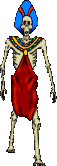5 Zombies (15, 5) - (14, 6) - (15, 6) - (18, 6) - (22, 22)

### Locations

• 12 Door openers (15, 10) - (17, 10) - (12, 11) - (20, 11) - (21, 14) - (23, 14) - (20, 15) - (24, 15) - (20, 17) - (24, 17) - (21, 18) - (23, 18)
• Alien Pipes-operated door opener (16, 10)
• 4 Do-not-disturb sectors(11, 19) - (10, 21) - (13, 21) - (28, 28)
• 3 Save runes(20, 22) - (5, 23) - (29, 30)
• False wall(18, 2) west side